# Leetcode 周赛No.124 笔记

## 993. 二叉树的堂兄弟节点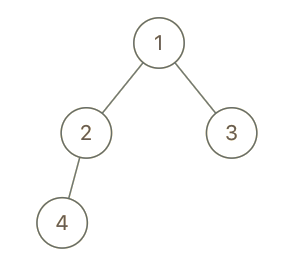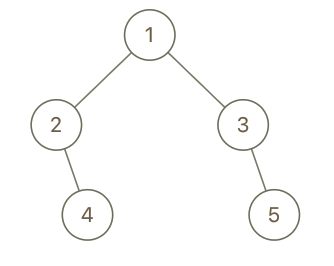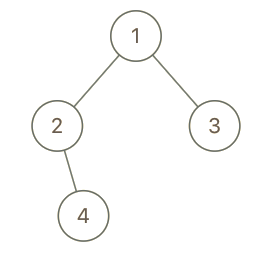1. 二叉树的节点数介于 2 到 100 之间。
2. 每个节点的值都是唯一的、范围为 1 到 100 的整数。

x、y是堂兄弟的两个条件：

1. x、y不同父。
2. x、y同层。

//my code
/**
* Definition for a binary tree node.
* public class TreeNode {
*     int val;
*     TreeNode left;
*     TreeNode right;
*     TreeNode(int x) { val = x; }
* }
*/
class Solution {
TreeNodePack root, xn, yn;
int x, y;
public boolean isCousins(TreeNode root, int x, int y) {
this.root = new TreeNodePack(root, 0, new TreeNode(-1));
this.x = x;
this.y = y;
this.dfs(this.root);
if(this.xn.depth == this.yn.depth && this.xn.father.val != this.yn.father.val)  //判断是不是堂兄弟
return true;
return false;
}
/* 深搜每个点并封装，遇到x和y点就提出来记录 */
public void dfs(TreeNodePack n){
if(n.n.val == this.x)
this.xn = n;
if(n.n.val == this.y)
this.yn = n;
if(n.n.left != null){
dfs(new TreeNodePack(n.n.left, n.depth + 1, n.n));
}
if(n.n.right != null){
dfs(new TreeNodePack(n.n.right, n.depth + 1, n.n));
}
}
}
/* 包装类 */
class TreeNodePack{
TreeNode n;  //包装点
int depth;  //它的层数
TreeNode father;  //它的父
TreeNodePack(TreeNode n, int depth, TreeNode father){
this.n = n;
this.depth = depth;
this.father = father;
}
}

//neal_wu's code
/**
* Definition for a binary tree node.
* struct TreeNode {
*     int val;
*     TreeNode *left;
*     TreeNode *right;
*     TreeNode(int x) : val(x), left(NULL), right(NULL) {}
* };
*/
class Solution {
public:
pair<int, int> x_info, y_info;

void dfs(TreeNode *node, int x, int y, int depth, int parent) {
if (node->val == x)
x_info = {depth, parent};

if (node->val == y)
y_info = {depth, parent};

if (node->left != nullptr)
dfs(node->left, x, y, depth + 1, node->val);

if (node->right != nullptr)
dfs(node->right, x, y, depth + 1, node->val);
}

bool isCousins(TreeNode *root, int x, int y) {
dfs(root, x, y, 0, -1);
return x_info.first == y_info.first && x_info.second != y_info.second;
}
};

## 994. 腐烂的橘子

• 0 代表空单元格；
• 1 代表新鲜橘子；
• 2 代表腐烂的橘子。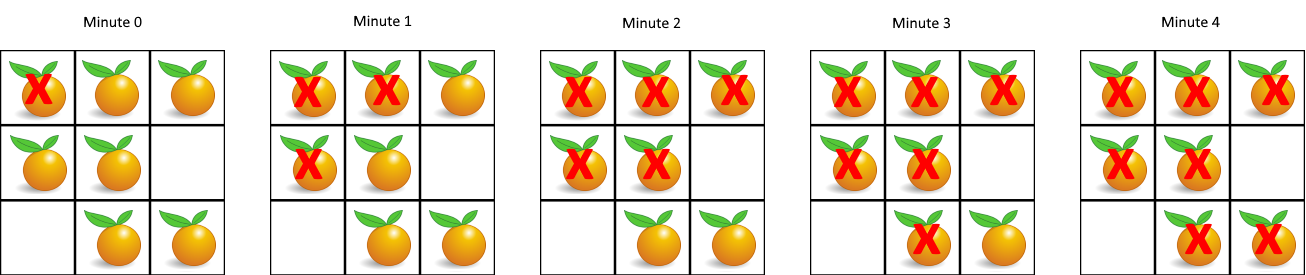1. 1 <= grid.length <= 10
2. 1 <= grid.length <= 10
3. grid[i][j] 仅为 0、1 或 2

1. 没有新鲜橘子了。
2. 即使有新鲜橘子也超过一定时间了（这个时间可以设置为长*宽）。
class Solution {
int[][] g;
public int orangesRotting(int[][] grid) {
this.g = grid;
int MAXMINUTE = 102;
int minute = 0;
for(int i=0; i<MAXMINUTE; i++){
if(this.getFreshAmount() == 0)
return minute;  //全烂了
this.relax();  //芝麻腐烂！
minute++;
}
return -1;
}
/* 执行一轮腐烂操作 */
public void relax(){
for(int i=0; i<g.length; i++){
for(int j=0; j<g.length; j++){
if(g[i][j] == 2){
if(i-1 >= 0 && g[i-1][j] == 1)
g[i-1][j] = 3;  //待腐烂状态
if(j-1 >= 0 && g[i][j-1] == 1)
g[i][j-1] = 3;
if(i+1 < g.length && g[i+1][j] == 1)
g[i+1][j] = 3;
if(j+1 < g.length && g[i][j+1] == 1)
g[i][j+1] = 3;
}
}
}
for(int i=0; i<g.length; i++){
for(int j=0; j<g.length; j++){
if(g[i][j] == 3)
g[i][j] = 2;
}
}
}
/* 获取当前有几个新鲜的 */
public int getFreshAmount(){
int ret = 0;
for(int i=0; i<g.length; i++){
for(int j=0; j<g.length; j++){
if(g[i][j] == 1)
ret++;
}
}
return ret;
}
}

## 995. K 连续位的最小翻转次数

1. 1 <= A.length <= 30000
2. 1 <= K <= A.length

//jasonshieh's code
class Solution {
public:
int minKBitFlips(vector<int>& A, int K) {
if(A.empty() || K <= 0) return -1;  //特殊值
int i = 0;
int n = A.size();
int res = 0;
while(i < n){
//略过数组前边一堆1
while(i < n && A[i] == 1){
i++;
}
//全为1的话就成了！
if(i == n) break;
//剩下的数不够K个就不可能翻成全1了！
if(n - i < K) return -1;
//如果能翻，从左往右数遇到的第一个0开始翻起，翻K个
for(int t = 0; t < K; ++t){
A[i+t] = (A[i+t] == 0 ? 1 : 0);
}
//步数+1
res++;
}
return res;
}
};

## 996. 正方形数组的数目

[1,8,17] 和 [17,8,1] 都是有效的排列。

1. 1 <= A.length <= 12
2. 0 <= A[i] <= 1e9

//nhho's code
//这个不知道是什么语言
/*
* 思路：有条件的递归遍历解法
*/
bool ok, u;
int n;
int x;
int ans;
set<int> s;
class Solution {
public:
/*
* 对数组进行递归形式的遍历，递归第a层表示的是这个排列的第a个项
* a：排列的第a项
* b：这个排列的第a项的值
* 排列完全按照题目要求进行排列，因此排完一次就是一个可行解
*/
void go(int a, int b) {
if (a == n)  //如果排到最后一项了就是可行解
ans++;
else
/*
* 否则对于每个剩余可行项尝试递归
* u[i]:记录i值是否在这个排列中用到了
*/
for (int i = 0; i < n; i++)
if (!u[i] && (b == -1 || ok[b][i]) && (i == 0 || x[i] != x[i - 1] || u[i - 1])) {
u[i] = 1;
go(a + 1, i);
u[i] = 0;
}
}
int numSquarefulPerms(vector<int>& A) {
sort(A.begin(), A.end());
//遍历2000000000以内平方数存入s
for (int i = 0; i * i <= 2000000000; i++)
s.insert(i * i);
//将A中每两个数相加看看是否是平方数,并将真值存入ok[][]
for (int i = 0; i < A.size(); i++)
for (int j = 0; j < A.size(); j++)
ok[i][j] = s.find(A[i] + A[j]) != s.end();
//刻印A的副本x
for (int i = 0; i < A.size(); i++)
x[i] = A[i];
ans = 0;
n = A.size();
go(0, -1);
return ans;
}
};

1.Yusorai说道：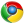Google Chrome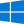Windows 10

可能这就叫做大佬吧 φ(￣∇￣o)

2.回味依旧说道：Google ChromeWindows 10

这么多人用你的软件竟然都没发现你的博客 我过来逛逛 嘿嘿嘿

3.文龙说道：Google Chrome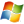Windows 7

发现软件更新下载好慢，然后就发现了这个博客